# HSSlive: Plus One & Plus Two Notes & Solutions for Kerala State Board

## AP Board Class 10 Physics Chapter 11 Electric Current Textbook Solutions PDF: Download Andhra Pradesh Board STD 10th Physics Chapter 11 Electric Current Book AnswersAP Board Class 10 Physics Chapter 11 Electric Current Textbook Solutions PDF: Download Andhra Pradesh Board STD 10th Physics Chapter 11 Electric Current Book Answers

## Andhra Pradesh State Board Class 10th Physics Chapter 11 Electric Current Books Solutions

 Board AP Board Materials Textbook Solutions/Guide Format DOC/PDF Class 10th Subject Maths Chapters Physics Chapter 11 Electric Current Provider Hsslive

2. Click on the Andhra Pradesh Board Class 10th Physics Chapter 11 Electric Current Answers.
3. Look for your Andhra Pradesh Board STD 10th Physics Chapter 11 Electric Current Textbooks PDF.

Find below the list of all AP Board Class 10th Physics Chapter 11 Electric Current Textbook Solutions for PDF’s for you to download and prepare for the upcoming exams:

### 10th Class Physics 11th Lesson Electric Current Textbook Questions and Answers

Question 1.
What do you mean by electric current?
(OR)
Define electric current.
Electric current is defined as the amount of charge crossing any cross-section of the conductor in one second.

Question 2.
Which type of charge (positive or negative) flows through an electric wire when it is connected in an electric circuit?
Negative type of charge flows through an electric wire when it is connected in an electric circuit.

Question 3.
Is there any evidence for the motion of charge in daily life situations?
Yes, lightning is a live example.

Question 1.
Explain how electron flow causes electric current with Lorentz – Drude theory of electrons. (AS1)
(OR)
How does electron flow cattle elfectric current with Lorentz – Drude theory of electrons? Explain.
Lorentz – Drude theory :

1. Lorentz – Drude proposed that conductors like metals contain a large number of free electrons.
2. The positive ions are fixed in their locations. The arrangement of the positive ions is called lattice.
3. The negative ions (electrons) move randomly in lattice in an open circuit.
4. When the lattice is closed the electrons are arranged in ordered motion.
5. When the electrons are in order motion, there will be a net charge (crossing through any cross section.
6. This order motion of electrons is called electric current.

Question 2.
How does a battery work? Explain. (AS1)
(OR)
How does a battery maintain a constant potential difference between its terminals?
Working of a battery :

• A battery consists of two metal plates (positive electrode = anode and negative electrode = cathode) and a chemical (electrolyte).
• The electrolyte between the two metal plates consists of positive and negative ions which move in opposite directions.
• The electrolyte exerts a chemical force on these ions and makes them move in a specified direction.
• Depending upon the nature of the chemical, positive ions move towards one of the plates and accumulate on that plate.
• As a result of this accumulation of charges on this plate it becomes anode.
• Negative ions move in a direction opposite to the motion of positive ions and accumulate on the other plate.
• As a result of this the plate becomes negatively charged called cathode.
• This accumulation of different charges on respective plates continues till both plates are sufficiently charged.
• But the ions in motion experience electric force when sufficient number of charges are accumulated on the plates.
• The motion of ions continues towards their respective plates till the chemical force is equal to electric force.
• Thus the battery works.

Question 3.
Write the difference between potential difference and emf. (AS1)
Potential Difference:
Work done by the electric force on unit charge is called potential difference.
𝐕=𝐖𝑞=𝐅𝑙𝐪

Electromotive force (emf):
The work done by the chemical force to move unit positive charge from negative terminal to positive terminal of the battery.
𝜀=𝑊𝑞=𝐹𝑑𝑞

Question 4.
How can you verify that the resistance of a conductor is temperature dependent? (AS1)
(OR)
How do you prove increase in temperature affects the resistance with an activity?
Resistance :
The resistance of a conductor is the obstruction offered to the flow of electrons in a conductor.

Resistance is temperature dependent:
Aim:
To show that the value of resistance of a conductor depends on temperature for constant voltage between the ends of the conductor.

Materials required :

1. A bulb
2. A battery
3. Key
4. Insulated wire
5. Multimeter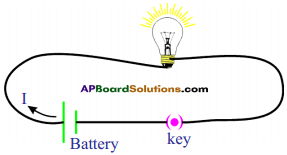Procedure :

1. Take a bulb and measure the resistance when it is in open circuit using a multimeter.
2. Note the value of resistance in your notebook.
3. Connect a circuit with components as shown in figure.
4. Switch on the circuit. After few minutes, measure the resistance of the bulb again.
5. Note this value in your notebook.

Observation :

1. The value of resistance of the bulb in second instance is more than the resistance of the bulb in open circuit.
2. The bulb gets heated.

Result:
The increase in temperature of the filament in the bulb is responsible for increase in resistance of the bulb.

Question 5.
What do you mean by electric shock? Explain how it takes place. (AS1)
Electric shock:
The electric shock is combined effect of potential difference, electric current, and resistance of the human body.

• An electric shock can be experienced when there exists a potential difference between one part of the body and another part.
• When current flows through human body, it chooses the path which offers low resistance.
• The resistance of a body is not uniform throughout it.
• As long as current flow continues inside the body, the current and resistance of human body go on changing inversely.
• This is called the electric shock.

Question 6.
Derive 𝐑=𝜌𝑙𝐀. (AS1)
(OR)
What are laws of resistance and derive a formula for resistance.
Resistance of a conductor is directly proportional to the length of the conductor,
i.e., R ∝ l ………………….. (1)
Resistance of a conductor is inversely proportional to the cross-section area of the conductor.
i.e., R ∝ 1 A ………………….. (2)
From (1) and (2) R ∝ 𝑅∝𝑙𝐴⇒𝑅=𝜌𝑙𝐴
where ρ is a constant,
ρ is called specific resistance or resistivity.

Question 7.
How do you verify that resistance of a conductor is proportional to the length of the conductor for constant cross-section area and temperature? (AS1)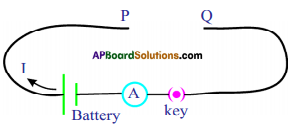• Collect manganin wires of different lengths with the same cross-sectional areas.
• Make a circuit as shown in figure.
• Connect one of the manganin wires between the ends P and Q.
•  Measure the value of the current using the ammeter.
• Repeat the same for other lengths of the wires.
• Note the values of currents.
• We notice that the current decreases with increase in the length of the wire.
∴ R ∝ l (at constant temperature and cross-section area) …………… (1)
• Do the same with manganin wires with equal lengths but different cross-section area.
• We notice that the resistance was more when the cross-section area was less.
∴ R ∝ 1 A ………………. (2)
∴ R ∝ [𝑙𝑎𝑡𝑒𝑥]1 A[/latex]
Thus we verify l and A.

Question 8.
Explain Kirchhoff’s laws with examples. (AS1)
(OR)
Write two examples of Kirchhoffs laws and explain it.
Kirchhoff’s laws :
Two simple rules called Kirchhoff’s rules are applicable to any DC circuit containing batteries and resistors connected in any way.
The two laws are (i) Junction law and (ii) Loop law.

i) Junction law :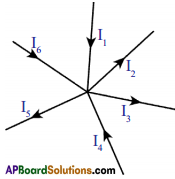Here P is called junction point where conducting wires meet. The junction law states that, at any junction point in a circuit where the current can divide, the sum of the currents into the junction must equal the sum of the currents leaving the junction.
i.e., I1 + I4 + I6 = I2 + I3 + I5
This law is based on the conservation of charge.

ii) Loop law: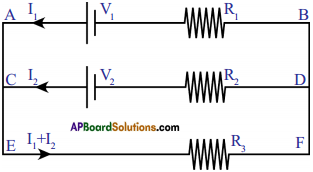Loop law states that, the algebraic sum of the increases and decreases in potential difference (voltage) across various components of the circuit in a closed circuit loop must be zero.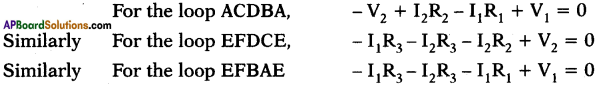This law is based on the conservation of energy.

Question 9.
What is the value of 1 KWH in Joules? (AS1)
1 KWH = 1 KW x 1h
= 1000 W × 60 min = 1000 W × 60 × 60 s = 3.6 × 106 Ws = 3.6 × 106 J.
∴ 1 KWH = 3.6 × 106 J.

Question 10.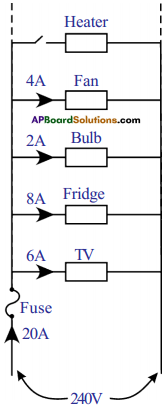• Electricity enters our homes through two wires called lines. These lines have low resistance and the potential difference between the wires is usually about 240 V.
• All electrical devices are connected in parallel in our home. Hence, the potential drop across each device is 240 V.
• Based on the resistance of each electric device, it draws some current from the supply. Total current drawn from the mains is equal to the sum of the currents passing through each device.
• If we add more devices to the household circuit the current drawn from the mains also increases.

Question 11.
Why do we use fuses in household circuits? (AS1)
(OR)
What is the use of fuses?

• The fuse consists of a thin wire of low melting point.
• When the current in the fuse exceeds 20 A, the wire will heat up and melt.
• The circuit then becomes open and prevents the flow of current into the household circuit.
• Hence all the electric devices are saved from damage that could be caused by overload.
• Thus we can save the household wiring and devices by using fuses.

Question 12.
Deduce the expression for the equivalent resistance of three resistors connected in series. (AS1)
(OR)
Derive R = R1 + R2 + R3
(OR)
The second end of a first resistor is connected to first end of second resistor. Then how are the resistors connected? Derive the expression for the resultant resistance of this connection.
Series connection:
In series connection of resistors, there is only one path for the flow of current in the circuit. Hence, the current in the circuit is equal to I.
According to Ohm’s law,
∴ V1 = IR1 ; V2 = IR2 and V3 = IR3.
⇒ Let R be the equivalent resistance of the combination of resistors in series.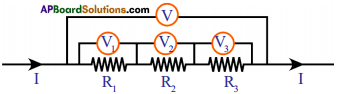Also V = I Req
V = V1 + V2 + V3
I Req = IR1 + IR2 + IR3
⇒ I Req = I (R1 + R2 + R3)
⇒ Req = R1 + R2 + R3
∴ The sum of individual resistances is equal to their equivalent resistance when the resistors are connected in series.

Question 13.
Deduce the expression for the equivalent resistance of three resistors connected in parallel. (AS1)
(OR)
Derive : 1𝐑=1𝐑1+1𝐑2+1𝐑3
(OR)
Explain the expression for the equivalent resistance of three resistors which are connected in parallel.
(OR)
If all the first ends of resistors are connected to and second ends are connected to another point, then what type of connection is this? Derive the resultant resistance for this connection.
Parallel Connection :
In parallel connection of resistors, there is same potential difference at the ends of the resistors. Hence the voltage in the circuit is equal to V.
Let Ip I2 and I3 be the currents flowing through R1, R2, and R3 resistors respectively.
Hence, we can write I = I1 + I2 + I3.
According to the Ohm’s law,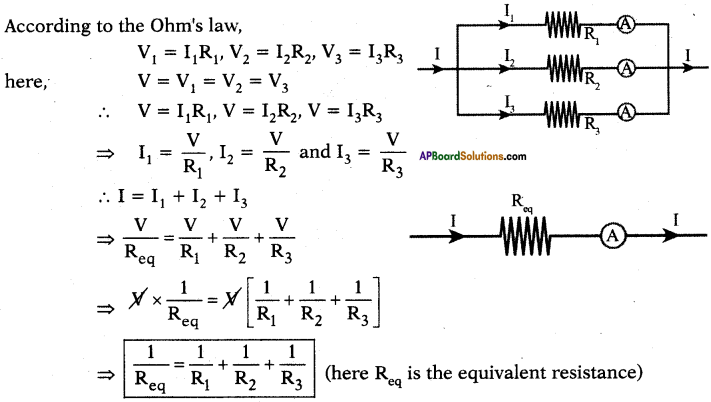∴ The equivalent resistance of a parallel combination is less than the resistance of each of the resistors.

Question 14.
Silver is a better conductor of electricity than copper. Why do we use copper wire for conduction of electricity? (AS1)
Silver is costlier than copper. So, we use copper wire for conduction of electricity even though silver is a better conductor of electricity.

Question 15.
Two bulbs have ratings 100 W, 220 V and 60 W, 220 V. Which one has the greater resistance? (AS1)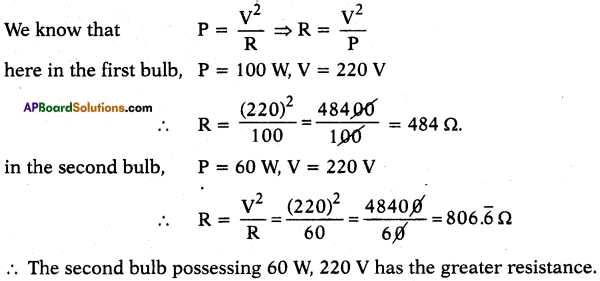∴ The second bulb possessing 60 W, 220 V has the greater resistance.

Question 16.
Why don’t we use series arrangement of electrical appliances like bulb, television, fan, and others in domestic circuits? (AS1)

• If one appliance, in a set of series combination breaks down, the circuit becomes open and the flow of current ceases. To avoid this the household appliances like bulb, T.V., fan, etc. are not connected in series. They are connected in parallel.
• In series combination same current passes through all resistors. This is not suggestable for household appliances. Hence, they are connected in parallel.

Question 17.
A wire of length 1 m and radius 0.1 mm has a resistance of 100 Ω. Find the resistivity of the material. (AS1)
1) Given l = 1 m, r = 0.1 mm = 10-4 m, R = 100 Ω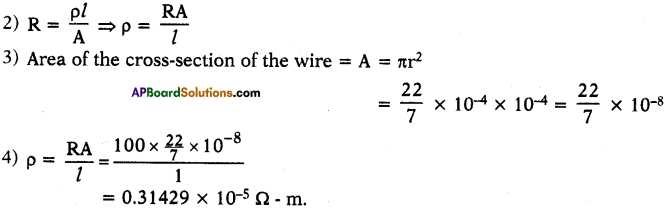Question 18.
Why do we consider tungsten as a suitable material for making the filament of a bulb? (AS2)
(OR)
What is the reason for using Tungsten as a filament in electric bulb?
Tungsten has higher resistivity values and melting point. So, we consider tungsten as a suitable material for making the filament of a bulb.

Question 19.
Are the head lights of a car connected in series or parallel? Why? (AS2)
The headlights of a car are connected in parallel.
Reason :

• When they are connected in parallel, same voltage (RD) will be maintained in the two lights.
• If one of the light damaged, the other will work without any disturbance.

Question 20.
Why should we connect electric appliances in parallel in a household circuit? What happens if they are connected in series?

• The electric appliances are connected in parallel in a household circuit. Because in parallel wiring if any electric appliance is switched off, other appliances don’t get off.
• If one appliance, in a set of series combination breaks down, the circuit becomes open and the flow of current ceases.
• To avoid this the household appliances like bulb, T.V., fan, etc. are not connected in series.

Question 21.
Suppose that you have three resistors each of value 30Ω. How many resistors can you obtain by various combinations of these three resistors? Draw diagrams in support of your predictions. (AS2)
Let R1 = 30Ω, R2 = 30Ω, R3 = 30Ω
We get different resistors by different combinations as shown below.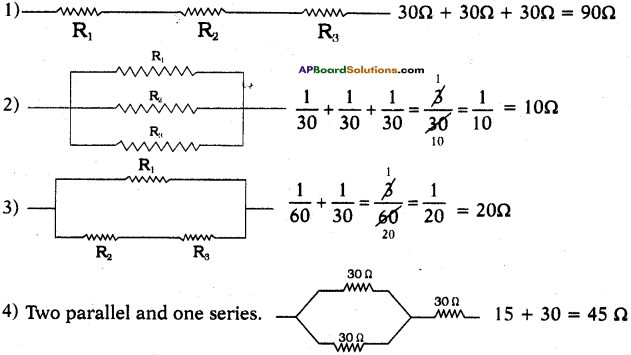Question 22.
State Ohm’s law. Suggest an experiment to verify it and explain the procedure. (AS3)
How do you prove experimentally the ratio V/l is a constant for a given conductor?
Ohm’s law :
The potential difference between the ends of a conductor is directly proportional to the electric current passing through it at constant temperature.

Verification :
Aim :
To verify Ohm’s law or to show that VI = constant for a conductor.

Materials required :
6V Battery eliminator, 0 to 1A Ammeter, 0 – 6V volt meter, copper wires, 50 cm manganin coil, Rheostat, switch and 3V LED, etc.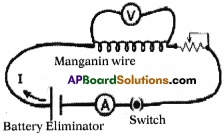Procedure :

• Complete the circuit as shown in figure. Knob should be adjusted to 4.5V at battery eliminator.
• Using Rheostat change the potential difference between two ends of manganin wire from 0V to 4.5V (maximum).
• By using Rheostat adjust the potential difference 1V between two ends of manganin wire.
• Now observe the electric current through Ammeter in the circuit and note down in the following table.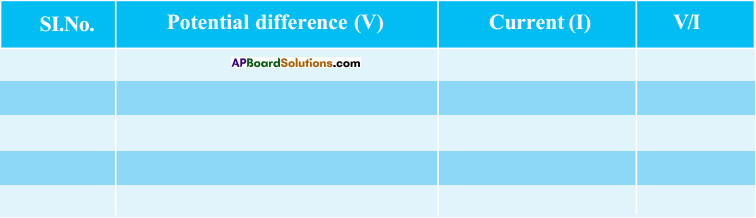• Using Rheostat change the potential difference with different values upto 4.5V and note down the current value (I) in the table.
• Take atleast five values of V and I and note down in the table.
• Find VI for each set of values.
• We notice that VI is a constant.
V ∝ I ⇒ VI = constant
This constant is known as resistance of the conductor, denoted by R.
⇒ VI = R
∴ Ohm’s law is verified.

How to Make Rheostat: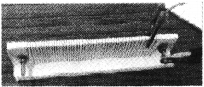Make two holes at the two ends of 30cm Wooden scale. Through these holes fix two bolts with the help of nuts.Then take iron box filament i. e., nichrome wire and tie one end of thewire to the first bolt and wound wire with equal distance on the wooden scale to other end of the second bolt. Place this scale on the other scale perpendicularly as shown in the figure and stick them with glue. Now Rheostat is ready. Take support of your teacher to know the connection and functioning of Rheostat.

Question 23.
a) Take a battery and measure the potential difference. Make a circuit and measure the potential difference when the battery is connected in the circuit. Is there any difference in potential difference of battery? (AS4)
b) Measure the resistance of a bulb (filament) in open circuit with a multi-meter. Make a circuit with elements such as bulb, battery of 12 V and key in series. Close the key. Then again measure the resistance of the same bulb (filament:) for every 30 seconds. Record the observations in a proper table. What can you conclude from the above results? (AS4)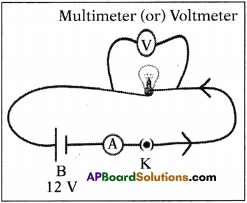a) When the battery is connected in a circuit, the voltage slowly decreases due to consumption of it. So, there is difference in voltage before using and after connecting.

b) After connecting battery (12 V), key in ammeter and bulb as shown in figure, we measure current (I) using the ammeter and voltage using multi-meter or voltmeter.

Note these values in the following table. Measure the resistance of the same bulb for every 30 seconds.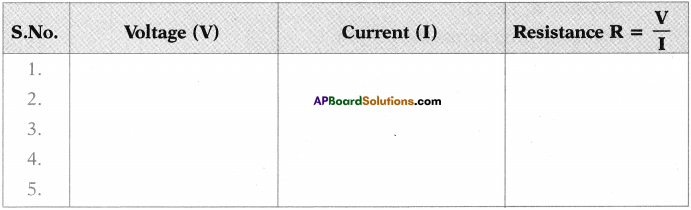We conclude that the resistance is constant.

Question 24.
Draw a circuit diagram for a circuit in which two resistors A and B are connected in series with a battery and a voltmeter is connected to measure the potential difference across the resistor A. (AS5)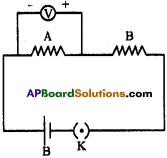V : Volt meter
A and B : Resistors
B : Battery
K: Key

Question 25.
How can you appreciate the role of a small fuse in house wiring circuit in preventing damage to various electrical appliances connected in the circuit? (AS7)
(OR)
We can save the household wiring and devices by using fuses. Write any four points by appreciating the role of fuse.

• The fuse consists of a thin wire of low melting point. When the current in the fuse exceeds 20 A, the wire will heat up and melt.
• The circuit then becomes open and prevents the flow of current into the household circuit. So all the electric devices are saved from damage that could be caused by overload.
• Thus we can save the household wiring and devices by using fuses.
• In this way a small fuse prevents a great damage to costly electrical appliances in the circuit.

Question 26.
In the figure, the potential at A is………….. when the potential at B is zero. (AS7)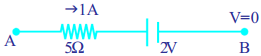Potential difference at A = V
Potential difference atB = V + 5 × 1 + 2 = 0 ⇒ V + 7V = 0
∴ V = – 7V

Question 27.
Observe the circuit and answer the questions given below. (AS7)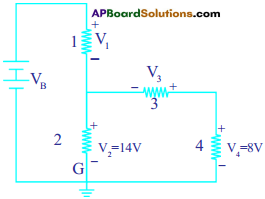i) Are resistors C and D in series?
ii) Are resistors A and B in series?
iii) Is the battery in series with.any resistor?
iv) What is the potential drop across the resistor C?
v) What is the total emf in the circuit if the potential drop across resistor A is 6 V?
The given circuit is written / drawn as
i) Yes, resistors ‘C’ and ‘D’ are connected in series. (Because, passing of the current is same to those resistors)
ii) No, resistors A’ and ‘B’ are not in series. (Because, different currents are passing through A and B. i.e., I1 and I2)
iii) The battery is in series with the resistor ‘A’. (Because, same current is passing through battery and resistor ‘A’, i.e., I)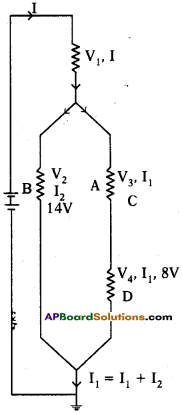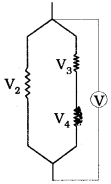iv) Potential drop across the resistor ‘C’
V2 = V3 + V4
14V = V3 + 8V
V3 = 6V
Potential drop = 6V

v) Total emf
emf of combination of V3 and V4 = 14V ……………….. (1)
emf of combination of (1) and V2 = 14 V ………………. (2)
emf of combination of (2) and V1 = 6V + 14V = 20V
(Given, emf of ‘A’ = 6V)
Total emf = 20V

Question 28.
If the resistance of your body is 100000 Cl, what would be the current that flows in your body when you touch the terminals of a 12 V battery? (AS7)
We know that, 𝐼=𝑉𝑅; here V = 12 V, R = 1,00,000Ω.
∴ The current passing through our body 𝐼=12 V100000Ω = 0.00012 Ampere.

Question 29.
A uniform wire of resistance 100 Ω is melted and recast into wire of length double that of the original. What would be the resistance of the new wire formed? (AS7)
Given R = 100 Ω
When ‘l = l’, R = 100 Ω.
When’l = 2l’, A’ = A / 2.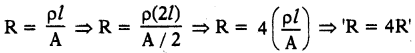∴ Resistance is increased by four times.
∴ R = 4 × 1ooΩ = 400Ω.

Question 30.
A house has 3 tube lights, two fans and a Television. Each tube light draws 40 W. The fan draws 80 W and the Television draws 60 W. On the average, all the tube lights are kept on for five hours, two fans for 12 hours and the television for five hours every day. Find the cost of electric energy used in 30 days at the rate of Rs. 3.00 per KWh. (AS7)
Given 3 tube lights, two fans and a television.
Power consumed by 1 tube light = 40 W
∴ Power consumed by 3 tube lights = 3 × 40W = 120W
3 tube lights are kept on for five hours. So, consumption of power by 3 tube lights
= 5 × 120 W = 600 W ……………. (1)
Power consumed by 1 fan = 80 W
∴ Power consumed by 2 fans = 2x80W=160W
2 fans are kept on for 12 hours. So, consumption of power by 2 fans
= 12 × 160 W = 1920 W ……………. (2)
Power drawn by TV = 60 W
TV is kept on for 5 hours = 5 x 60 W = 300 W ………………. (3)
∴ Consumption of power in one day = (1) + (2) + (3)
= 600W+ 1920 W + 300 W = 2820 W = 2.820 KW
∴ Total consumption of power in 30 days at Rs. 3 per KW
= 2.820 × 30 × 3 = Rs. 253.80/-

Fill in The Blanks

1. The kilowatt hour is the unit of …………………..
2. A thick wire has ………………….. resistance than a thin wire.
3. An unknown circuit draws a current of 2 A from a 12 V battery. Its equivalent resistance is …………………..
4. The SI unit of potential difference is …………………..
5. The SI unit of current is …………………..
6. Three resistors of values 2Ω, 4Ω, 6Ω are connected in series. The equivalent resistance of combination of resistors is ……………………
7. Three resistors of values 2Ω, 4Ω, 6Ω are connected in parallel. The equivalent resistance of combination of resistors is ……………………
8. The power delivered by a battery of emf, 10 V is 10 W. Then the current delivered by the battery is ……………………

1. electrical energy
2. less
3. 6 Ω
4. volt
5. Ampere
6. 12 Ω
7. 1112Ω
8. 1 ampere

Multiple Choice Questions

1. A uniform wire of resistance 50 Ω. is cut into five equal parts. These parts are now connected in parallel. Then the equivalent resistance of the combination is
A) 2 Ω
B) 12 Ω
C) 250 Ω
D) 6250 Ω
A) 2 Ω

2. A charge is moved from a point A to a point B. The work done to move unit charge during this process is called
A) potential at A
B) potential at B
C) potential difference between A and B
D) current from A to B
C) potential difference between A and B

3. Joule/ coulomb is the same as
A) 1 – watt
B) 1 – volt
C) 1- ampere
D) 1 – ohm
B) 1 – volt

4. The current in the wire depends
A) only on the potential difference applied
B) only on the resistance of the wire
C) on potential difference and resistance
D) none of them
C) on potential difference and resistance

5. Consider the following statements.
a) In series connection, the same current flows through each element.
b) In parallel connection, the same potential difference gets applied across each element
A) both a and b are correct
B) a is correct but b is wrong
C) a is wrong but b is correct
D) both a and b are wrong
A) both a and b are correct

### 10th Class Physics 11th Lesson Electric Current InText Questions and Answers

10th Class Physics Textbook Page No. 179

Question 1.
Does motion of charge always lead to electric current?
Yes, it does.

Question 2.
Take a bulb, a battery, a switch and few insulated copper wires to the terminals of the battery through the bulb and switch. Now switch on the circuit and observe the bulb. What do you notice?
The bulb glows.

10th Class Physics Textbook Page No. 180

Question 3.
Can you predict the reason for the bulb not glowing in situations 2 and 3?
Yes, in situation 2 – there is no charge to travel in the circuit as the battery is disconnected. So, the bulb isn’t glowing.

In situation 3, we replaced the copper wires with nylon wires. Nylon is not a conductor. So, the bulb isn’t glowing.

Question 4.
Why do all materials not act as conductors?
In conductors the gap between the atoms is very less. So, the transfer of energy is easy. But in other materials the gap is more. So, the transfer of energy is not possible.

Question 5.
How does a conductor transfer energy from source to bulb

• A source has chemical energy which transfers electrons to the conductor.
• The conductor carries the electrons to the bulb when connected.
• Thus, the conductor transfers energy from source to bulb.

Question 6.
What happens to the motion of electrons when the ends of the conductor are connected to the battery?
The energy transfer takes place from battery to the bulb through conductor.

10th Class Physics Textbook Page No. 181

Question 7.
Why do electrons move in specified direction?
The electrons move in specified direction when the ends of the conductpr are connected to the terminals of a battery.
A uniform electric field is set up throughout the conductor. This field makes the electrons move in a specified direction.

Question 8.
In which direction do the electrons move?
In a direction opposite to the direction of the field.

Question 9.
Do the electrons accelerate continuously?
No, they lose energy and are again accelerated by the electric field.

Question 10.
Do they move with a constant speed?
Yes, they move with a constant average Speed.

Question 11.
Why does a bulb glow immediately when we switch on?
When we switch on any electric circuit, irrespective of length of the conductor, an electric field is set up throughout the conductor instantaneously due to the voltage of the source connected to the circuit.

Question 12.
How can we decide the direction of electric current?
By the signs of the charge and drift speed.

10th Class Physics Textbook Page No. 183

Question 13.
How can we measure electric current?
An ammeter is used to measure electric current.

Question 14.
Where do the electrons get energy for their motion from?
From an electric field set up throughout the conductor.

Question 15.
Can you find the work done by the electric force?
Yes. With the help of the formula W = Fel, we can find the work done by the electric force.

Question 16.
What is the work done by the electric force on unit charge?
Work done by the electric force on unit charge V=Wq=Fe𝑙q. It is called Potential difference.

10th Class Physics Textbook Page No. 184

Question 17.
What is the direction of electric current in terms of potential difference?
Electrons move from low potential to high potential.

Question 18.
Do positive charges move in a conductor? Can you give an example of this?
No, they don’t move. They are fixed in the lattice.
Eg : battery.

Question 19.
How does a battery maintain a constant potential difference between its terminals?
We know that a battery consists electric force (Fe) and chemical force (Fc). These two forces are balanced in a battery. Due to this reason a battery maintains a constant , potential difference between its terminals.

Question 20.
Why does the battery discharge when its positive and negative terminals are connected through a conductor?
A conductor permits the charges to pass through it. Due to this the exhaustion of charges is created after completion of all charges. So, when a battery is connected with a conductor it discharges.

10th Class Physics Textbook Page No. 185

Question 21.
What happens when the battery is connected in a circuit?
A potential difference is created between the ends of the conductor, when the battery is connected in a circuit.

10th Class Physics Textbook Page No. 186

Question 22.
How can we measure potential difference or emf?
With the help of a voltmeter, we measure potential difference or emf.

10th Class Physics Textbook Page No. 187

Question 23.
Is there any relation between emf of battery and drift speed of electrons in the conductor connected to a battery?
Yes, when emf of a battery is more the drift speed of electrons will be more.

10th Class Physics Textbook Page No. 189

Question 24.
Can you guess the reason why the ratio of V and I in case of LED is not constant?
This is due to forward voltage and maximum continuous current rating characters of LEDs.

Question 25.
Do all materials obey Ohm’s law?
No, some materials don’t obey Ohm’s law.

Question 26.
Can we classify the materials based on Ohm’s law?
Yes, the materials which obey Ohm’s law are conductors and others are same conductors or non-conductors.

Question 27.
What is resistance?
The obstruction offered to the flow of electrons in a conductor is called the resistance.

Question 28.
Is the value of resistance the same for all materials?
Yes, it varies.

Question 29.
Is there any application of Ohm’s law in daily life?
Yes, this law is used in wiring.

Question 30.
What causes electric shock in the human body – current or voltage?
Current with sufficient voltage.

10th Class Physics Textbook Page No. 190

Question 31.
Do you know the voltage of mains that we use in our household circuits?
Yes, I know the voltage of mains that we use in our household circuits is 120 V.

Question 32.
What happens to our body if we touch live wire of 240 V?
240 V current disturbs the functioning of organs inside the body. It is called electric shock. If the current flow continues further, it damages the tissues of the body which leads to decrease in resistance of the body. When this current flows for a longer time, damage to the tissues increases and thereby the resistance of human body decreases further. Hence, the current through the human body will increase. If this current reaches 0.07 A, it effects the functioning of the heart and if this much current passes through the heart for more than one second it could be fatal.

If this current flows for a longer time, the person in electric shock will be killed.

10th Class Physics Textbook Page No. 191

Question 33.
Why doesn’t a bird get a shock when it stands on a high voltage wire?
There are two parallel lines carrying 240 V current. The voltage current will pass through the body if both the wires are touched at the same time. But, when the bird stands on only one wire, there is no potential difference between the legs. So, no current passes through the bird. Hence, it doesn’t feel any electric shock.

10th Class Physics Textbook Page No. 192

Question 34.
What could be the reason for increase in the resistance of the bulb when current flows through it?
The increase in temperature of the filament in the bulb is responsible for increase in resistance of the bulb.

Question 35.
What happens to the resistance of a conductor if we increase its length?
The resistance of a conductor increases with the increase of its length.

10th Class Physics Textbook Page No. 193

Question 36.
Does the thickness of a conductor influence its resistance?
Yes, as the thickness of the conductor increases the resistance decreases.

10th Class Physics Textbook Page No. 195

Question 37.
How are electric devices connected in circuits?
Electric devices are connected either in series or parallel in circuits.

Question 38.
When bulbs are connected (resistors) in series, what do you notice
We notice that, the sum of the voltages of the bulbs (resistors) is equal to voltage across the combination of the resistors.

10th Class Physics Textbook Page No. 196

Question 39.
What do you notice when bulbs (resistors) are connected in series to the current?
The current is not changing

Question 40.
What do you mean by equivalent resistance?
If the current drawn by a resistor is equal to the current drawn by the combination of resistors, then the resistor is called equivalent resistor.

Question 41.
What happens when one of the resistors in series breaks down?
The circuit becomes open and flow of current will be broken down.

Question 42.
Can you guess in what way household wiring has been done?
Parallel connection.

10th Class Physics Textbook Page No. 197

Question 43.
How much current is drawn from the battery if the resistors are connected in parallel?
Is it equal to individual currents drawn by the resistors?
It is the sum of currents flowing through each resistor. No, it is the sum of individual currents drawn by the resistors.

10th Class Physics Textbook Page No. 199

Question 44.
How could the sign convention be taken in a circuit?
The potential difference across the resistor is taken as negative when we move along the direction of electric current through the resistor, and it is taken as positive when we move against the direction of electric current through the resistor.

10th Class Physics Textbook Page No. 201

Question 45.
You might have heard the sentences like “this month we have consumed 100 units of current”. What does ‘unit’ mean?
Unit (or) kilo watt hour is the consumption of electric power in one hour by our electric appliances.

Question 46.
A bulb is marked 60 W and 120 V. What do these values indicate?
It means, the resistance of the bulb is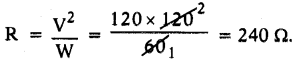Question 47.
What is the energy lost by the charge in 1 sec.?
It is equal to Wt.

10th Class Physics Textbook Page No. 202

Question 48.
What do you mean by overload?
When a high current flows through the wire which is beyond the rating of wire then heating of wire takes place. This phenomenon is called overloading.

Question 49.
Due to overload the heat increases in the circuit and this melts the parts of the appliances. Thus overload causdt damage to the electric appliances.

10th Class Physics Textbook Page No. 203

Question 50.
What happens when this current (overloading) increases greatly to the household circuit?
It causes fire.

Question 51.
To prevent damages due to overloading we connect an electric fuse to the household circuit.

10th Class Physics Textbook Page No. 203

Question 52.
What do you mean by short circuit?

• The line wires that are entering the meter have a voltage of 240 V.
•  The minimum and maximum limit of current that can be drawn from the mains is 5 to 20 A.
• Thus, the maximum current that we can draw from the mains is 20 A.
• When the current drawn from the mains is more than 20 A, overheating occurs and may cause a fire. This is called overloading.
• A short circuit is an electrical circuit that allows a current to travel along an unintended path often where essentially no electrical impedance is encountered.

Question 52.
Why does a short circuit damage electric wiring and devices connected to it?
In a short circuit the current drawn from the main exceeds the maximum limit 20 A. This will lead to overloading which can damage the electrical appliances.

10th Class Physics 11th Lesson Electric Current Activities

Activity – 1

Question 1.
Write an activity to check when a bulb glows in a circuit.
(OR)
How do you prove a source of energy is required to glow a bulb in a circuit?
Aim :
To check when a bulb glows in a circuit.

Materials required:

1. A bulb
2. a battery
3. a switch
4. few insulated copper wire

Procedure (1) :

1. Take a bulb, a battery, a switch and few insulated copper wires.
2. Connect the ends of the copper wires to the terminals of the battery through the bulb and switch.
3. Now switch on the circuit.
Observation (1) : The bulb glows.

Procedure (2) :

1. Remove the battery from the circuit and connect the remaining components to make a complete circuit.
2. Again switch on the circuit and observe the bulb.

Observation (2): The bulb does not glow.

Procedure (3) :
Replace the copper wires with nylon wires and connect the nylon wires to the terminals of the battery through a bulb and switch. Now switch on the circuit. We observe that the bulb does not glow. Because the wires are not conductors.

Observation (3) : The bulb does not glow.

Result:
The battery contains charges which glow the bulb.

Activity – 3

Question 2.
Write an activity to show that the values of current are different for different wires for a constant voltage.
(OR)
The resistance of a conductor depends on the material of the conductor. Prove this through an activity.
(OR)
List out the material required in the experiment to show that the electric resistance depends upon the nature of the material and write experimental procedure.
Aim:
To show that the values of current are different for different wires for a constant voltage. Materials required : (wires of the same length and some cross-sectional area).

• Copper rod
• Nichrome rod
• Battery
• Ammeter
• Key
• Manganin Wire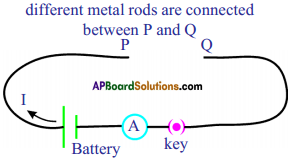Procedure :

1. Make a circuit as shown in figure.
2. Connect one of the wires between the ends P and Q.
3. Switch on the circuit. Measure the electric current for a fixed voltage, using the ammeter connected to the circuit. Note it in your notebook.
4. Repeat this experiment with other wires and note the current in your notebook.

Observation :
The values of current are different for different wires for a constant voltage.

Conclusion:
The resistance of a conductor depends on the material of the conductor.

Activity – 5

Question 3.
Write an activity to show that resistance is inversely proportional to the c section area of the conductor.
(OR)
What happens to resistance if the area of a cross-section of conductor is increased? Explain with an activity.
Aim :
To prove that resistance is inversely proportional to the cross-section area of the conductor.

Materials required :

1. A Battery
2. Mangnin Wires
3. Ammeter
4. Key
5. Manganin wires with different cross-section areas (lengths are same).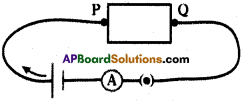Procedure:

1. Make the circuit as given figure.
2. Connect one of the wires between points P and Q.
3. Switch on me circuit. Note the ammeter reading in your notebook.
4. Continue the experiment with different wires of same length but different cross-section areas. Note the ammeter readings in your notebook.

Observation :
As the cross-section area of the rods increases, the current increases.

Result (Conclusion) :
Resistance is inversely proportional to cross-section area of the conductor.

Activity – 6

Question 4.
Write an activity to prove that the sum of the potential differences of the bulb is equal to voltage across the combination of the resistors. (OR)
Prove that during series connection potential difference is distributed among the resistors experimentally.
Aim:
To prove that the sum of the potential differences of the bulbs is equal to potential difference across the combination of the resistors.

Materials required :

1. Bulbs
2. Voltmeters
3. Insulated wires
4. Ammeter
5. Key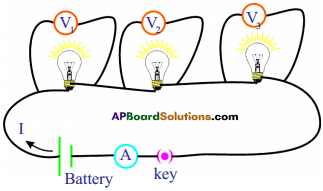Procedure :

1. Take different bulbs. Using a multimeter measure their resistances. Note them as R,, R2 and Rv
2. Connect them as shown in figure.
3. Measure the voltage between terminals of the battery connected to the circuit.
4. Measure the voltages between the ends of each bulb and note them as Vj, V2 and V3 from voltmeters in your notebook.
5. Compare them.

Observation :
We notice that the’sum of the voltages of the bulbs is equal to voltages across the combination of the resistors.

Activity – 7

Question 5.
Write an activity to prove that the current drawn from the battery is equal to the sum of individual currents drawn by the bulbs.
(OR)
Prove that during parallel connection the current is distributed among the resistances by using an experimental activity.
Aim:
To prove that the current drawn from the battery is equal to the sum of individual currents drawn by the bulbs.
Materials required :

1. Bulbs
2. Ammeters
3. Buttery
4. Key
5. Wires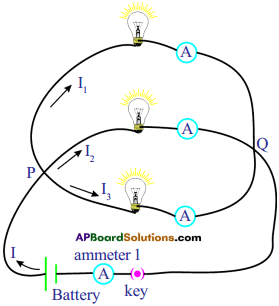Procedure :

1. Connect the bulbs in parallel connection as shown in the given circuit.
2. Measure the voltage across each bulb using a voltmeter or multimeter.
3. Note these values in your notebook.

Observation :

1. The voltage at the ends of each bulb is the same.
2. Measure electric currents flowing through each bulb using ammeters. Note these values.
3. Measure the current (I) drawn from the battery using the ammeter 1.

Result (Conclusion) :
The current drawn from the battery is equal to the sum of individual currents drawn by the bulbs.

## Andhra Pradesh Board Class 10th Physics Chapter 11 Electric Current Textbooks for Exam Preparations

Andhra Pradesh Board Class 10th Physics Chapter 11 Electric Current Textbook Solutions can be of great help in your Andhra Pradesh Board Class 10th Physics Chapter 11 Electric Current exam preparation. The AP Board STD 10th Physics Chapter 11 Electric Current Textbooks study material, used with the English medium textbooks, can help you complete the entire Class 10th Physics Chapter 11 Electric Current Books State Board syllabus with maximum efficiency.

## FAQs Regarding Andhra Pradesh Board Class 10th Physics Chapter 11 Electric Current Textbook Solutions

#### Can we get a Andhra Pradesh State Board Book PDF for all Classes?

Yes you can get Andhra Pradesh Board Text Book PDF for all classes using the links provided in the above article.

## Important Terms

Andhra Pradesh Board Class 10th Physics Chapter 11 Electric Current, AP Board Class 10th Physics Chapter 11 Electric Current Textbooks, Andhra Pradesh State Board Class 10th Physics Chapter 11 Electric Current, Andhra Pradesh State Board Class 10th Physics Chapter 11 Electric Current Textbook solutions, AP Board Class 10th Physics Chapter 11 Electric Current Textbooks Solutions, Andhra Pradesh Board STD 10th Physics Chapter 11 Electric Current, AP Board STD 10th Physics Chapter 11 Electric Current Textbooks, Andhra Pradesh State Board STD 10th Physics Chapter 11 Electric Current, Andhra Pradesh State Board STD 10th Physics Chapter 11 Electric Current Textbook solutions, AP Board STD 10th Physics Chapter 11 Electric Current Textbooks Solutions,
Share: